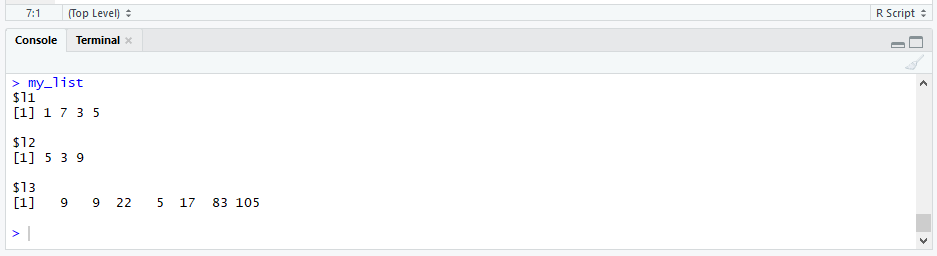# R unlist Function | 3 Example Codes (List of Vectors, Data Frame & String)

Basic R Syntax:

`unlist(my_list)`

The unlist R function converts a list to a single vector. The basic code for unlist in R is illustrated above.

In the following tutorial, I will show you several examples for the application of the unlist function in R. Let’s dive in…

## Example 1: Unlist List of Vectors in R

Let’s begin with a simple example: The conversion of a list of numeric vectors into a single vector. Consider the following example list:

```my_list <- list(l1 = c(1, 7, 3, 5), # First list element l2 = c(5, 3, 9), # Second list element l3 = c(9, 9, 22, 5, 17, 83, 105)) # Third list element my_list```Graphic 1: Example List for the Application of unlist in R.

Our example list consists of three elements. Each of them is a numeric vector.

Now, let’s apply the unlist R function:

```unlist(my_list) # Apply unlist R function # l11 l12 l13 l14 l21 l22 l23 l31 l32 l33 l34 l35 l36 l37 # 1 7 3 5 5 3 9 9 9 22 5 17 83 105```

As you can see based on this unlist R example, the function returns a vector consisting of all values of the three vectors within my_list.

Additionally, each value of the output vector is named with the list name + an indicator for the position of each value within the original list element. For instance, the value 7 appears in the first list element l1 at the second position. For that reason, in the output vector its name is l12 (i.e. l1 + 2).

If you want to get rid of these names, you can simply add the specification use.names = FALSE within the unlist R function:

```unlist(my_list, use.names = FALSE) # Apply unlist without names # 1 7 3 5 5 3 9 9 9 22 5 17 83 105```

Check out the following video of my YouTube channel. The video explains the R programming syntax of Example 1 in more detail:

## Example 2: Unlist a List with Data Frames

The unlist function can also be applied to a list that includes a data frame. Consider the following modified example list:

```my_list_2 <- my_list # Create modified list my_list_2[] <- data.frame(x1 = c(5, 1, 2), # Add a data frame to the list x2 = c(7, 5, 7))```

To such a list with dataframes, we can also apply the unlist command:

```unlist(my_list_2) # Unlist list with data.frame # l11 l12 l13 l14 l21 l22 l23 l31 l32 l33 l34 l35 l36 l37 x11 x12 x13 x21 x22 x23 # 1 7 3 5 5 3 9 9 9 22 5 17 83 105 5 1 2 7 5 7```

As you can see, each column of the data matrix is unlisted itself.

## Example 3: Unlist a String in R

It is even possible to unlist a string (i.e. a data object of character class). First, let’s modify our list once more:

```my_list_3 <- my_list_2 # Create modified list my_list_3[] <- c("A", "Y", "H") # Add string element to list```

Our new list, my_list_3, consists of three numeric vectors, one data table of the class data.frame, and one character vector.

Now, let’s apply the unlist command to this list:

```unlist(my_list_3) # Unlist string in R # l11 l12 l13 l14 l21 l22 l23 l31 l32 l33 l34 l35 l36 l37 # "1" "7" "3" "5" "5" "3" "9" "9" "9" "22" "5" "17" "83" "105" # x11 x12 x13 x21 x22 x23 # "5" "1" "2" "7" "5" "7" "A" "Y" "H"```

No problem – Everything is converted into one single vector. However, note that all values of the output vector are converted to the character class!

## Video Examples: The unlist Function Explained

Have a look at the following video of the YouTube channel R Programming Tips. The video provides you with more examples of unlist in R.